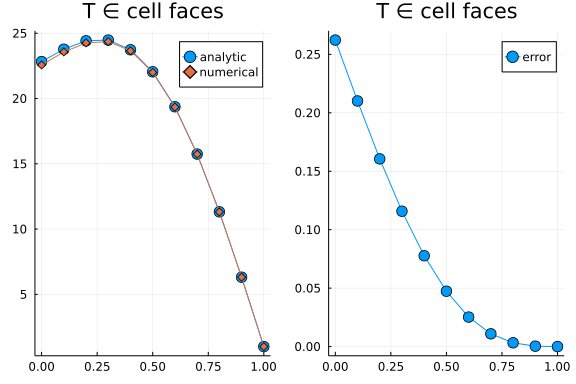# Solving the heat equation with diffusion-implicit time-stepping

##### Charles Kawczynski

In this tutorial, we'll be solving the heat equation:

$∂_t T = α ∇²(T) + β \sin(γ z)$

with boundary conditions: $∇T(z=a) = ∇T_{bottom}, T(z=b) = T_{top}$. We'll solve these equations numerically using Finite Difference Method on cell faces. The same exercise could easily be done on cell centers.

First, we'll use / import some packages:

import Plots
using LinearAlgebra
using DiffEqBase
using OrdinaryDiffEq: SplitODEProblem, solve, IMEXEuler
import SciMLBase


Next, we'll define some global problem parameters:

a,b, n = 0, 1, 10               # zmin, zmax, number of cells
n̂_min, n̂_max = -1, 1            # Outward facing unit vectors
α = 100;                        # thermal diffusivity, larger means more stiff
β, γ = 10000, π;                # source term coefficients
Δt = 1000;                      # timestep size
N_t = 10;                       # number of timesteps to take
FT = Float64;                   # float type
Δz = FT(b-a)/FT(n)
Δz² = Δz^2;
∇²_op = [1/Δz², -2/Δz², 1/Δz²]; # interior Laplacian operator
∇T_bottom = 10;                 # Temperature gradient at the top
T_top = 1;                      # Temperature at the bottom
S(z) = β*sin(γ*z)               # source term, (sin for easy integration)
zf = range(a, b, length=n+1);   # coordinates on cell faces


## Derivation of analytic solution

Here, we'll derive the analytic solution:

$\frac{∂²T}{∂²z} = -\frac{S(z)}{α} = -\frac{β \sin(γ z)}{α} \\ \frac{∂T}{∂z} = \frac{β \cos(γ z)}{γ α}+c_1 \\ T(z) = \frac{β \sin(γ z)}{γ^2 α}+c_1 z+c_2, \qquad \text{(generic solution)}$

Apply bottom boundary condition:

$\frac{∂T}{∂z}(a) = \frac{β \cos(γ a)}{γ α}+c_1 = ∇T_{bottom} \\ c_1 = ∇T_{bottom}-\frac{β \cos(γ a)}{γ α}$

Apply top boundary condition:

$T(b) = \frac{β \sin(γ b)}{γ^2 α}+c_1 b+c_2 = T_{top} \\ c_2 = T_{top}-\left(\frac{β \sin(γ b)}{γ^2 α}+c_1 b\right)$

And now let's define this in a julia function:

function T_analytic(z) # Analytic steady state solution
c1 = ∇T_bottom-β*cos(γ*a)/(γ*α)
c2 = T_top-(β*sin(γ*b)/(γ^2*α)+c1*b)
return β*sin(γ*z)/(γ^2*α)+c1*z+c2
end

T_analytic (generic function with 1 method)


## Derive the temporal discretization

Here, we'll derivation the matrix form of the temporal discretization we wish to use (diffusion-implicit and explicit Euler):

$∂_t T = α ∇²T + S \\ (T^{n+1}-T^n) = Δt (α ∇²T^{n+1} + S) \\ (T^{n+1} - Δt α ∇²T^{n+1}) = T^n + Δt S \\ (I - Δt α ∇²) T^{n+1} = T^n + Δt S$

Note that, since the $∇²$ reaches to boundary points, we'll need to modify the stencils to account for boundary conditions.

## Derive the finite difference stencil

For the interior domain, a central and second-order finite difference stencil is simply:

$∇²f = \frac{f_{i-1} -2f_i + f_{i+1}}{Δz²}, \qquad \text{or} \\ ∇² = \left[\frac{1}{Δz²}, \frac{-2}{Δz²}, \frac{1}{Δz²}\right] \\$

At the boundaries, we need to modify the stencil to account for Dirichlet and Neumann BCs. Using the following index denotion:

• i first interior index

• b boundary index

• g ghost index

the Dirichlet boundary stencil & source:

$∂_t T = α \frac{T[i-1]+T[b]-2 T[i]}{Δz²} + S \\ ∂_t T = α \frac{T[i-1]-2 T[i]}{Δz²} + S + α \frac{T[b]}{Δz²}$

and Neumann boundary stencil & source:

$∇T_{bottom} n̂ = \frac{T[g] - T[i]}{2Δz}, \qquad n̂ = [-1,1] ∈ [z_{min},z_{max}] \\ T[i] + 2 Δz ∇T_{bottom} n̂ = T[g] \\ ∂_t T = α \frac{\frac{(T[i] + 2 Δz ∇T_{bottom} n̂) - T[b]}{Δz} - \frac{T[b] - T[i]}{Δz}}{Δz} + S \\ ∂_t T = α \frac{\frac{T[i] - T[b]}{Δz} - \frac{T[b] - T[i]}{Δz}}{Δz} + S + α 2 Δz \frac{∇T_{bottom}}{Δz²} \\ ∂_t T = α \frac{2 T[i] - 2 T[b]}{Δz²} + S + 2α \frac{∇T_{bottom} n̂}{Δz}$

## Define the discrete diffusion operator

# Initialize interior and boundary stencils:
∇² = Tridiagonal(
ones(FT, n) .* ∇²_op,
ones(FT, n+1)   .* ∇²_op,
ones(FT, n) .* ∇²_op
);

# Modify boundary stencil to account for BCs

∇².d = -2/Δz²
∇².du = +2/Δz²

# Modify boundary stencil to account for BCs
∇².du[n] = 0  # modified stencil
∇².d[n+1] = 0 # to ensure ∂_t T = 0 at z=zmax
∇².dl[n] = 0  # to ensure ∂_t T = 0 at z=zmax
D = α .* ∇²

11×11 LinearAlgebra.Tridiagonal{Float64, Vector{Float64}}:
-20000.0   20000.0        ⋅         ⋅   …        ⋅         ⋅         ⋅
⋅
10000.0  -20000.0   10000.0        ⋅            ⋅         ⋅         ⋅
⋅
⋅    10000.0  -20000.0   10000.0           ⋅         ⋅         ⋅
⋅
⋅         ⋅    10000.0  -20000.0           ⋅         ⋅         ⋅
⋅
⋅         ⋅         ⋅    10000.0           ⋅         ⋅         ⋅
⋅
⋅         ⋅         ⋅         ⋅   …        ⋅         ⋅         ⋅
⋅
⋅         ⋅         ⋅         ⋅       10000.0        ⋅         ⋅
⋅
⋅         ⋅         ⋅         ⋅      -20000.0   10000.0        ⋅
⋅
⋅         ⋅         ⋅         ⋅       10000.0  -20000.0   10000.0
⋅
⋅         ⋅         ⋅         ⋅            ⋅    10000.0  -20000.0  0
.0
⋅         ⋅         ⋅         ⋅   …        ⋅         ⋅        0.0  0
.0


## Define boundary source

Here, we'll compute the boundary source $\left(\frac{α T[b]}{Δz²}\right)$

AT_b = zeros(FT, n+1);
AT_b = α*2/Δz*∇T_bottom*n̂_min;
AT_b[end-1] = α*T_top/Δz²;


## Set initial condition

Let's just initialize the solution to 1, and also set the top boundary condition:

T = zeros(FT, n+1);
T .= 1;
T[n+1] = T_top; # set top BC


## Define right-hand side sources

Here, we define the right-hand side (RHS) sources:

function rhs!(dT, T, params, t)
n = params.n
i = 1:n # interior domain
dT[i] .= S.(zf[i]) .+ AT_b[i]
return dT
end;


Next, we'll pacakge up parameters needed in the RHS function, define the ODE problem, and solve.

params = (;n)

tspan = (FT(0), N_t*FT(Δt))

prob = SplitODEProblem(
SciMLBase.DiffEqArrayOperator(
D,
),
rhs!,
T,
tspan,
params
)
alg = IMEXEuler(linsolve=LinSolveFactorize(lu!))
println("Solving...")
sol = solve(
prob,
alg,
dt = Δt,
saveat = range(FT(0), N_t*FT(Δt), length=5),
progress = true,
progress_message = (dt, u, p, t) -> t,
);

Solving...


# Visualizing results

Now, let's visualize the results of the solution and error:

T_end = sol.u[end]

p1 = Plots.plot(zf, T_analytic.(zf), label="analytic", markershape=:circle, markersize=6)
p1 = Plots.plot!(p1, zf, T_end, label="numerical", markershape=:diamond)
p1 = Plots.plot!(p1, title="T ∈ cell faces")

p2 = Plots.plot(zf, abs.(T_end .- T_analytic.(zf)), label="error", markershape=:circle, markersize=6)
p2 = Plots.plot!(p2, title="T ∈ cell faces")

Plots.plot(p1, p2)